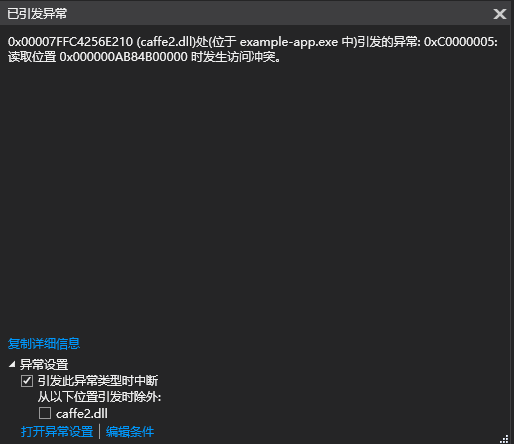# How to speed up Conversion from C++ STL data to Tensor?

(Yi Liu) #1

I am doing some optimization on NMS, I write it by Cython.
since I have to compare it with original one, so I try to write a pytorch C++ extension, I need INT to index, while Variable cannot do this, so I use Vector to restore result, but it seems that C++ extension needs a torch format data to return, and I have to convert Vector to Tensor. I tried torch:: from_blob, it runs fast but will be wrong after return as the pointer’s data has been released. if I get an INT point from an empty tensor and give it value one by one from the vector, it will be much slower, any idea to speed up it?
Cython can return vector as python list, so I am wondering can pytorch C++ extension do this too?
I also need to do a comparison in the GPU environment and taking data from GPU seems to cost a lot of time too. Is there any optimization can do on this?
Thanks for any help and ideas !!! (°:з」∠)

(Thomas V) #2

The options here seem to be to either hold on to the data or to use `from_blob(...).clone()`.

Best regards

Thomas

(Yi Liu) #3

I tried your suggestion, however, I find using clone() is slower than giving value one by one, here is my test code:

``````#include <torch/torch.h>
#include <iostream>
#include <vector>
#include <typeinfo>
#include <ctime>
#include <opencv2/core/core.hpp>
#include "opencv2/imgproc.hpp"
#include<opencv2/highgui/highgui.hpp>
#include<windows.h>
using namespace std;
int main() {
LARGE_INTEGER nFreq;
LARGE_INTEGER start, end;int length = 200000;
int class_num = 5;
vector<vector<float>> test_vector;
vector<float> tmp_h_list;
for (int i = 0; i < length; ++i) {
tmp_h_list.clear();
for (int j = 0; j < class_num; ++j) {
tmp_h_list.push_back(i*j);
};
test_vector.push_back(tmp_h_list);
};
QueryPerformanceFrequency(&nFreq);
QueryPerformanceCounter(&start);
torch::Tensor y = torch::zeros({ length, class_num }, torch::kFloat32);
QueryPerformanceCounter(&end);
cout <<"init y: "<< (double)(end.QuadPart - start.QuadPart)/ (double)nFreq.QuadPart *1000 << "ms" << endl;
QueryPerformanceCounter(&start);
torch::Tensor yc = torch::clone(y);
QueryPerformanceCounter(&end);
cout << "clone y: " << (double)(end.QuadPart - start.QuadPart) / (double)nFreq.QuadPart * 1000 << "ms" << endl;
QueryPerformanceCounter(&start);
float* tmp_ptr = y.data<float>();
for (int i = 0; i < length; ++i) {
for (int j = 0; j < class_num; ++j) {
*tmp_ptr++ = test_vector[i][j];
};
};
QueryPerformanceCounter(&end);
cout << "clone one by one: " << (double)(end.QuadPart - start.QuadPart) / (double)nFreq.QuadPart * 1000 << "ms" << endl;
system("pause");
}
``````

result is

``````init y: 2.28433ms
clone y: 2.98833ms
clone one by one: 2.05215ms
``````

I also find there will be a error when trying to clone a Tensor created by from_blob(<vector<…>>*):
Exception thrown at 0x00007FFC4256E210 (caffe2.dll) (in example-app.exe): 0xC0000005: An access violation occurred while reading location 0x000000AB84B00000.Thanks a lot for your time(Kirill) #4

You can’t pass pointer to type the `vector<vector<float>>` to the torch::from_blob function, because it assumes that you pass pointer to contiguous memory, so you can pass only something like `float*`. There is `vector<T>::data()` method in STL.

(Yi Liu) #5

Got it, Thanks a lot！